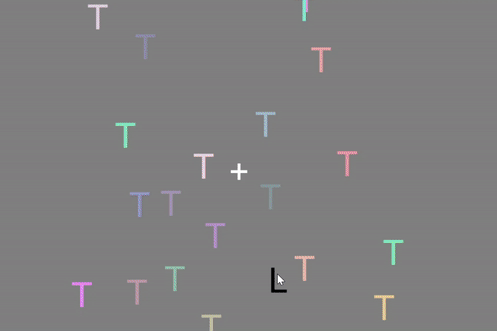# Making many components (a visual search task)¶

## Why would we need to “make in mass” ?¶

Imagine we are making a visual search task, where there is one target (the letter “L”) amongst a lorge number of letter “T”s. We could make one component for each letter, but depending on the size of our search array that could get tricky, and mean alot of manual labour in the long run (e.g. if we wanted to change the size of each letter, we would have to change the letter height in all the components)….

We have already learnt how to draw something with code when we learnt about coding experiments from scratch. This can now be used in builder view by using what we learnt in code components!

## Exporting builder to code¶

Remember, we can export a builder experiment to coder at any point, but we emphasised this is a one-way street and highly recommend avoiding editing the underlying python file. But exporting a python file can be useful to study how to do something in python if we are not sure. Start by adding a simple textStim with the letter “T” in the Text field, call the component “myLetter”. Save it and export the python file then search for “myLetter”.

Hopefully you can see under the hood how to make a textStim component:

```myLetter = visual.TextStim(win=win, name='myLetter',
text='T',
font='Open Sans',
pos=(0, 0), height=0.1, wrapWidth=None, ori=0.0,
color='white', colorSpace='rgb', opacity=None,
languageStyle='LTR',
depth=0.0))
```

Note

When we use these “pinched” bits of python code they might not always translate smoothly to Javascript. If this happens, export your experiment to Javascript and instead use the Javascript version of the component.

## Making many stimuli¶

We have learnt about how to use loops to add to lists, Objects created from components can be treated the same as any other variable i.e. we can have a list of Objects (also known as “instances” in python - in python lingo, here we have an “instance” of the “TextStim” class).

To make a list of textStim, we could use:

```myLetters = []

for i in range(10):
myLetter = visual.TextStim(win=win, name='myLetter',
text='T',
font='Open Sans',
pos=(0, 0), height=0.1, wrapWidth=None, ori=0.0,
color='white', colorSpace='rgb', opacity=None,
languageStyle='LTR',
depth=0.0)

myLetters.append(myLetter)
```

This would make a list of 10 letters, but because they would all be in the same position, we would only see one letter if we drew them.

To present stimuli in a set of positions we could do something like this:

```myXList = [-.5, 0, .5]
myLetters = []

for x in myXList:
myLetter = visual.TextStim(win=win, name='myLetter',
text='T',
font='Open Sans',
pos=(x, 0), height=0.1, wrapWidth=None, ori=0.0,
color='white', colorSpace='rgb', opacity=None,
languageStyle='LTR',
depth=0.0)
myLetters.append(myLetter)

print(myLetters)
```

This time we would have 3 letters but each would have a different X position, so we would be able to see them if we draw them.

## Drawing many stimuli¶

We have already learnt that all classes in the visual module have a method called `draw()` and `setAutoDraw()` and we know that the second of these methods means the object is drawn every time the window flips untill we declare `setAutoDraw(False)`.

Add a routine called “search” and add a fixation point that lasts the duration of our search time, let’s give it 10 seconds. OK then we need a code component, let’s call it ‘drawAll’. In the’Begin Routine’ tab, type:

```for letter in myLetters:
letter.setAutoDraw(True)
```

Then in the ‘End Routine’ tab type:

```for letter in myLetters:
letter.setAutoDraw(False)
```

If you run your experiment, you should now see three letter T’s displayed onscreen.

## making a list of random positions¶

OK so this works, but with three positions we might think “what’s the point”, let’s make this worth our while by making 20 letters all at random locations. Rather than using a fixed set of positions let’s change our code to the following:

```myLetters = []

for x in range(20):
myLetter = visual.TextStim(win=win, name='myLetter',
text='T',
font='Open Sans',
pos=(random(), random()), height=0.1, wrapWidth=None, ori=0.0,
color='white', colorSpace='rgb', opacity=None,
languageStyle='LTR',
depth=0.0)
myLetters.append(myLetter)
```

Here the method `random()` is used to generate a random value between 0 and 1.

Note

`random()` is a python method, if you are working online you will need the Javascript equivilent which is `Math.random()` (hopefully psychopy will do this for you soon though!)

Because these random values are all positive, you might notice all the letters are in the top right hand side of the screen, so let’s scale those positions to be `pos=(random()-0.5, random()-0.5)`.

Finally, let’s add a target, because there is only one of these we can use a basic component!

## Exercise (20 mins)¶

1. Make all of the distractors random colors.

Hint: remember the color field of a text stim can take rgb values e.g. [1, 0, 0] would be red

1. Add a mouse that can click on the target.

2. Make the trial repeat 5 times.

3. Make the target appear in random locations on each trial.

4. Make the number of distractors change on each trial…

The end product should look like this…